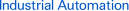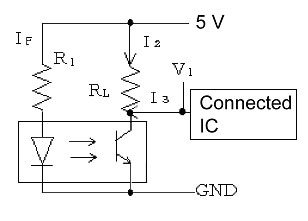Page top# FAQ01127 of Photomicro Sensors FAQ

FAQ No. FAQ01127

Question

The output voltage of one Phototransistor is much higher than others. What's causing this and what can be done?

﻿

Cause:
There may be an error in the circuit constant setting. Specifically, the output voltage may be high because the load resistance connected to the receiver's phototransistor is too small.

Countermeasure: Set an appropriate load resistance value to correct the circuit constant.

Refer to the following example of incorrect operation with EE-SX1041 Photomicrosensor due to an error in setting the load resistance and the method for correcting error by calculating an appropriate load resistance value.

Reference Explanation:

Model: EE-SX1041

Example Name: Incorrect Operation Due to Error in Setting Load Resistance Values

Example description and cause: The load resistance value is too small, so the phototransistor cannot pass the circuit current.

(Optical current IL < Circuit current I2 + I3, so the phototransistor cannot pass the circuit current, and output V11 does not drop below the voltage to be detected, L.)

Example: Using the EE-SX1041 with the Following CircuitCircuit constants

R1 = 220Ω, RL = 10 kΩ

Specifications of Connected IC

Current I3 flowing from IC when input voltage is low: 0.1 mA

The following is a detailed explanation of the calculation. R1 = 220 M, so LED forward current IF = (VCC − VF)/R1 = (5 − 1.2)/220 = 17 mA. (Formula 1)

For the EE-SX1041, the standard value of light current IL = 0.5 to 14 mA (when IF = 20 mA). If IL is found using proportional calculation, IL = 0.425 to 11.9 mA (when IF = 17 mA). (Formula 2)

And, circuit current I2 + I3 = (VCC − VCE(sat))/RL + I3 = (5 − 0.1)/10 k + 0.1 = 0.59 mA. (Formula 3).

The relationship of the values found using formulas 2 and 3 is

IL = 0.425 mA < I2 + I3 = 0.59 mA, so the phototransistor will not pass the circuit current and the output will not reach L if the mounted phototransistor is mounted near the specified lower limit of light current IL (in this case, near 0.5 mA when IF = 20 mA).

(There is variation in the sensor light current IL, so even if there are no problems during the prototype stage, variations in the Sensor after starting mass production may result in incorrect operation. Always perform calculations and check that there will be no problem even if the Sensor is near the specified lower limit of the light current IL.)

Correction Procedure:

(For the circuit above, set the load resistance RL to approximately 47 kΩ.)

Calculation Procedure:

Generally design the Photomicrosensor system anticipating a 50% reduction from the initial value of IL considering degradation over time and temperature characteristics.

Accordingly, the settings must be made so that IL x 0.5 > I2 + I3.

Calculating using the calculation example above,

IL = 0.425 mA x 0.5 > I2 + I3

0.212 > I2 + I3

0.212 > (VCC − VCE(sat))/RL + 0.1

RL > ((5 − 0.1)/(0.212 − 0.1)) = 43.8k Ω ,so

RL = Approximately 47 kΩ.

## Recommended Products

###E2EStandard Sensors for Detecting Ferrous Metals under Standard Conditions